• ## 概率密度分布

千次阅读 2015-12-31 15:45:39
参数密度估计大多数实际应用过程中，缺乏概率分布的具体解析式，因此需要通过所观察到的样本进行进行估计，通常分为： 参数估计 非参数估计 参数估计 已知：概率分布的解析表达式 求解：确定表达式中的参数 主要方法...
参数密度估计

大多数实际应用过程中，缺乏概率分布的具体解析式，因此需要通过所观察到的样本进行进行估计，通常分为：

参数估计
非参数估计
参数估计

已知：概率分布的解析表达式
求解：确定表达式中的参数
主要方法

最大似然估计（频率学派）
最大后验概率估计（多个先验，贝叶斯学派）
贝叶斯推理
最大熵模型
混合模型（EM算法）
最大似然估计

算法

    1）把参数当做未知实数（不是变量） ------频率学派
2）需要解析式，很多时候是困难的。解析式是正态分布时，等同于最小二乘法。


随机样本x1,x2,...,xN<!--//--><![CDATA[//><!--
x_1,x_2,...,x_N
//--><!]]>来自概率分布p(x;θ)<!--//--><![CDATA[//><!--
p(x;\theta)
//--><!]]>
假设样本对立，则联合概率: p(X;θ)=p(x1,x2,...,xN;θ)=∏k=1Np(xk;θ)<!--//--><![CDATA[//><!--
p(X;\theta) = p(x_1,x_2,...,x_N;\theta)=\prod_{k=1}^Np(x_k;\theta)
//--><!]]>
最大似然方法(max likelihood)估计使似然函数取最大值（一般来说先取对数，方便计算） θ¯ML=argmaxθ∏k=1Kp(xk;θ)<!--//--><![CDATA[//><!--
\bar{\theta}_{ML} = argmax_\theta \prod_{k=1}^Kp(x_k;\theta)
//--><!]]>
导数为零，进行求解。（或者其他有效的最优化技术,如梯度下降算法等）。
∂∏Kk=1p(xk;θ)∂θ=0<!--//--><![CDATA[//><!--
\frac{\partial\prod_{k=1}^Kp(x_k;\theta)}{\partial\theta}=0
//--><!]]>     多数的方程会不可导，可以查阅相关资料进行最优化


具体细节

一般去对数似然函数，大多数也是这么做的，求解方便（如高斯公式取对数） L(θ)=ln∏k=1Np(xk;θ)<!--//--><![CDATA[//><!--
L(\theta) = ln \prod_{k=1}^Np(x_k;\theta)
//--><!]]>∂L(θ)∂θ=∑k=1N∂lnp(xk;θ)∂θ=∑k=1N1p(xk;θ)∂p(xk;θ)∂θ=0<!--//--><![CDATA[//><!--
\frac{\partial L(\theta)}{\partial \theta}
= \sum_{k=1}^N \frac{\partial lnp(x_k;\theta)}{\partial \theta}
=\sum_{k=1}^N \frac{1}{p(x_k;\theta)} \frac{\partial p(x_k;\theta)}{\partial \theta}=0
//--><!]]>我们可以看出，原来需要对整个连乘公式求偏导，现在需要为每个概率公式求偏导，然后累加。许多公式本身求偏导是有很多良好性质（logister等），计算很简便。

性质

渐进无偏估计（无偏性，根据定义证明即可）
渐进一致的
渐进高效的，最小方差值
参数估计的质量

我们假设有一个理想的模型（我们的目标，也是模式识别的基础），但是目前手里只有一些抽样出来的样本。我们可以认为，学习出来的模型是基于少量样本的，但是我们追求的是适用于所有样本的模型。

当前模型估计的越复杂，那么在该数据集上（目前训练集上）准确性越好，那么模型的鲁棒性（在所有样本上）就会有所下降
当前模型估计的越简单，那么在该数据集上准确性就有所下降，但是模型的鲁棒性会相对上升。


我们不知道目前数据集的可靠性，做的太好（过分推理，过拟合），在测试集上就不一定表现的很好。做的太差（欠拟合，从训练样本中，学习了很少），在测试集上表现平平。这边说的做的太好，做的太差，当然是相对于训练集的。
因此，到底要做怎么样的平衡，通常就是借助验证集来评判。

偏差：真实值与估计值的距离（训练集上的表现）
方差：对于不同数据集，参数估计值的变化
对于高斯分布而言，我们很容易证明：

均值估计是无偏的
方差估计是有偏的（样本很大的时候，偏差消失。因为，当样本很大，先验作用开始不明显，也就是频率学认为先验来自于样本。或者可以从贝叶斯公式也能看出。）
最大后验概率估计

算法

最大后验概率认为θ<!--//--><![CDATA[//><!--
\theta
//--><!]]>是随机变量。而不是一个未知参数。（两派之争）
后验概率: p(θ|X),X=x1,x2,...,xN<!--//--><![CDATA[//><!--
p(\theta|X), X = {x_1,x_2,...,x_N}
//--><!]]>
根据贝叶斯定理求出后验概率，然后最大化后验概率（MAP） p(θ|X)=p(X|θ)p(θ)p(X)<!--//--><![CDATA[//><!--
p(\theta|X)=\frac{p(X|\theta)p(\theta)}{p(X)}
//--><!]]>
导数为零，进行求解。（或者其他有效的最优化技术）。 ∂p(θ|X)∂θ=0或者∂p(X|θ)p(θ)∂θ=0<!--//--><![CDATA[//><!--
\frac{\partial p(\theta|X)}{\partial \theta}=0
或者
\frac{\partial p(X|\theta)p(\theta)}{\partial \theta}=0
//--><!]]>
讨论

主要差别在于先验（当先验相同时，或者差别不大时，其差别很小）
贝叶斯推理

算法

已知N<!--//--><![CDATA[//><!--
N
//--><!]]>个样本集合X<!--//--><![CDATA[//><!--
X
//--><!]]>和先验概率P(θ)<!--//--><![CDATA[//><!--
P(\theta)
//--><!]]>,计算条件概率密度函数p(x|X)<!--//--><![CDATA[//><!--
p(x|X)
//--><!]]>
p(x|X)=∫p(x|θ)p(θ|X)dθ<!--//--><![CDATA[//><!--
p(x|X) = \int p(x|\theta)p(\theta|X)d\theta
//--><!]]>其中

p(θ|X)=p(X|θ)p(θ)p(X)=p(X|θ)p(θ)∫p(X|θ)p(θ)dθ<!--//--><![CDATA[//><!--
p(\theta|X) = \frac{p(X|\theta)p(\theta)}{p(X)}=\frac{p(X|\theta)p(\theta)}{\int p(X|\theta)p(\theta)d\theta}
//--><!]]>p(X|θ)=∏k=1Np(xk|θ)<!--//--><![CDATA[//><!--
p(X|\theta) = \prod_{k=1}^N p(x_k|\theta)
//--><!]]>最大熵

    熵是系统不确定性的度量


定义： H=−∫xp(x)lnp(x)dx<!--//--><![CDATA[//><!--
H = - \int_x p(x)ln p(x) dx
//--><!]]>在具体应用是，只需要看看概率密度函数需要满足那些条件即可。

若均值和方差作为其它约束，对于，概率密度函数的最大熵估计为高斯分布

混合模型

线性组合表示p(x)<!--//--><![CDATA[//><!--
p(x)
//--><!]]>p(x)=∑j=1Jp(x|j)Pj<!--//--><![CDATA[//><!--
p(x) = \sum_{j=1}^J p(x|j)P_j
//--><!]]>其中需要满足一些概率性质（和为1） ∑j=1JPj=1,∫xp(x|j)dx=1<!--//--><![CDATA[//><!--
\sum_{j=1}^J P_j = 1  ,  \int_x p(x|j) dx = 1
//--><!]]>选取合适，可以近似任何连续的密度函数

准备工作

选取相关密度组成函数 p(x|j)<!--//--><![CDATA[//><!--
p(x|j)
//--><!]]>,也就是p(x|j;θ)<!--//--><![CDATA[//><!--
p(x|j;\theta)
//--><!]]>
根据观察样本，求解相关参数θ,Pj<!--//--><![CDATA[//><!--
\theta,P_j
//--><!]]>
方法

最大化似然函数

∏kp(xk;θ,P1,P2,...,PJ)<!--//--><![CDATA[//><!--
\prod_k p(x_k;\theta,P_1,P_2,...,P_J)
//--><!]]>难以求解最大值，本质上缺少标签信息，无监督的问题。EM算法可以求解此类问题。

EM算法

有监督学习问题中，概率密度函数为py(y;θ)<!--//--><![CDATA[//><!--
p_y(y;\theta)
//--><!]]>
那么在无监督中， px(x;θ)=∫Y(x)py(y;θ)dy<!--//--><![CDATA[//><!--
p_x(x;\theta) = \int_{Y(x)} p_y(y;\theta) dy
//--><!]]>
最大似然估计 ∑k∂lnpy(yk;θ)∂θ=0<!--//--><![CDATA[//><!--
\sum_k \frac {\partial lnp_y(y_k;\theta) }{\partial \theta} = 0
//--><!]]>
EM 在观察样本和θ<!--//--><![CDATA[//><!--
\theta
//--><!]]>当前估计的条件下最大化似然函数的期望
算法流程
1）计算期望 Q(θ;theta(t))=E[∑kln(py(yk;θ|X;;θ(t)))]<!--//--><![CDATA[//><!--
Q(\theta;theta(t)) = E[\sum_k ln(p_y(y_k;\theta|X;;\theta(t)))]
//--><!]]>
2)最大化 ∂Q(θ;θ(t))∂θ=0<!--//--><![CDATA[//><!--
\frac{\partial Q(\theta;\theta(t))}{\partial \theta}=0
//--><!]]>    似然函数一直在不断增大，直至收敛


讨论

假设有标签 p(xk，jk;θ)=p(xk|jk;θ)Pjk<!--//--><![CDATA[//><!--
p(x_k，j_k;\theta) = p(x_k|j_k;\theta)P_{jk}
//--><!]]>
似然 L(θ)=∑k=1Nln(p(xk|jk;θ)Pjk)<!--//--><![CDATA[//><!--
L(\theta) = \sum_{k=1}^N ln(p(x_k|j_k;\theta)P_{jk})
//--><!]]>
上课笔记 
展开全文• 概率密度分布函数是不同事件发生的概率，自变量是样本取值，这样说可能不便于理解，下边通过二项分布概率公式说明： 上边是二项分布计算概率的一般公式，似然函数中的自变量是公式中的p，而概率密度分布函数中的自...
似然函数是某一特定事件发生的概率，其中自变量是分布参数θ，特定事件（一组样本取到一组特定值的联合概率）发生的概率随θ的不同而不同
概率密度分布函数是不同事件发生的概率，自变量是样本取值，这样说可能不便于理解，下边通过二项分布概率公式说明：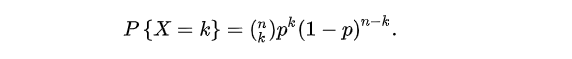上边是二项分布计算概率的一般公式，似然函数中的自变量是公式中的p，而概率密度分布函数中的自变量是公式中的k
如果你还是不理解，这里引用quora上的一个回答  What is the difference between probability and likelihood

我们可以再做一个类比，假设一个函数为 a^b ，这个函数包含两个变量。 如果你令b=2，这样你就得到了一个关于a的二次函数，即  ：a^2；当你令a=2时，你将得到一个关于b的指数函数，即  2^b
可以看到这两个函数有着不同的名字，却源于同一个函数。而p(x|θ)也是一个有着两个变量的函数。如果，你将θ设为常量，则你会得到一个概率函数（关于x的函数）；如果，你将x设为常量你将得到似然函数（关于θ的函数）。


展开全文似然函数 极大似然估计 概率密度函数
• 累积分布函数(Cumulative Distribution Function)，又叫分布函数，是概率密度函数的积分，能完整描述一个实随机变量X的概率分布。一般以大写CDF标记,，与概率密度函数probability density function（小写pdf）相对。...
累积分布函数(Cumulative Distribution Function)，又叫分布函数，是概率密度函数的积分，能完整描述一个实随机变量X的概率分布。一般以大写CDF标记,，与概率密度函数probability density function（小写pdf）相对。即累积分布函数表示：对离散变量而言，所有小于等于a的值出现概率的和.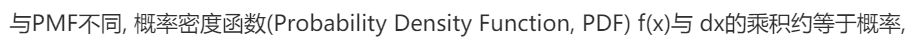可以把概率密度看成是纵坐标，区间看成是横坐标，概率密度对区间的积分就是面积，而这个面积就是事件在这个区间发生的概率，所有面积的和为1。
所以单独分析一个点的概率密度是没有任何意义的，它必须要有区间作为参考和对比。


展开全文• x=-3:0.2:3;...%泊松分布概率密度作图： x=0:20; y1=poisspdf(x,2.5);%以2.5 y2=poisspdf(x,5);%以5 y3=poisspdf(x,10);%以10 hold on plot(x,y1,':r*') plot(x,y2,':b*') plot(x,y3,':g*') hold off
x=-3:0.2:3;
y=normpdf(x,0,1);
plot(x,y)
hold on;
%正态
%累积
x=-3:0.2:3;
y=normcdf(x,0,1);
plot(x,y)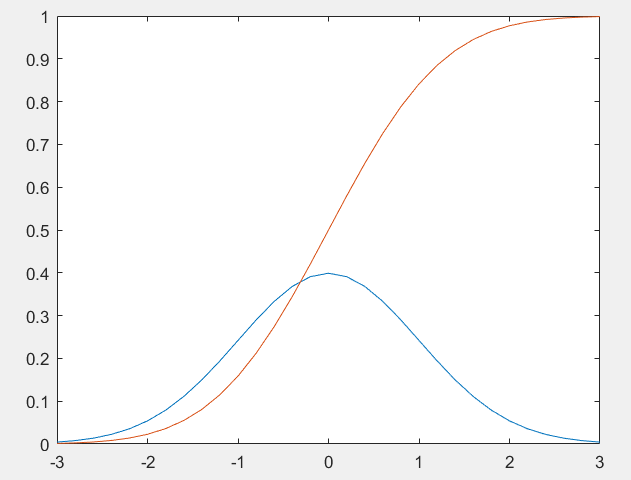%泊松分布概率密度作图：
x=0:20;
y1=poisspdf(x,2.5);%以2.5
y2=poisspdf(x,5);%以5
y3=poisspdf(x,10);%以10
hold on
plot(x,y1,':r*')
plot(x,y2,':b*')
plot(x,y3,':g*')
hold off
title('Poisson分布')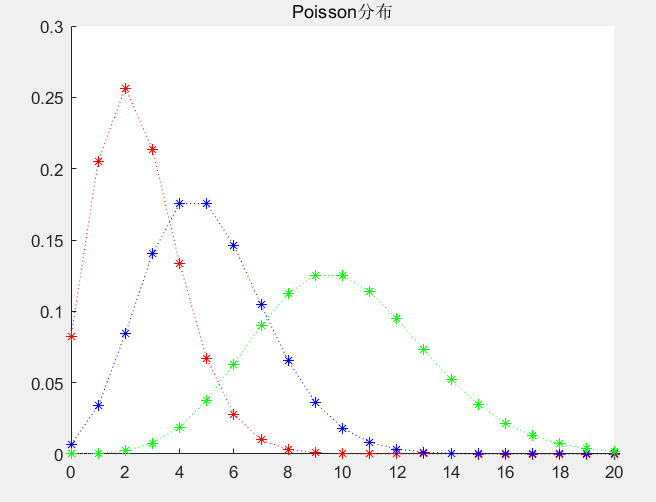y=rand(1,3000);
ymin=min(y);
ymax=max(y);
x=linspace(ymin,ymax,20);%将最大最小区间分成20个等分点(19等分),然后分别计算各个区间的个数
yy=hist(y,x);%计算各个区间的个数
yy=yy/length(y);%计算各个区间的个数(比率)
bar(x,yy)%画出概率密度分布图

s=0;
for i=2:length(x)
s=[s,trapz(x([1:i]),yy([1:i]))];   % please remove the " ; "
end
figure;
plot(x,s,x,s,'*')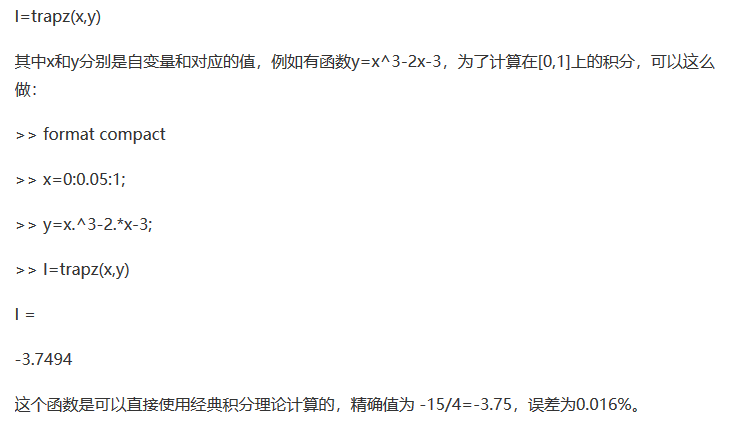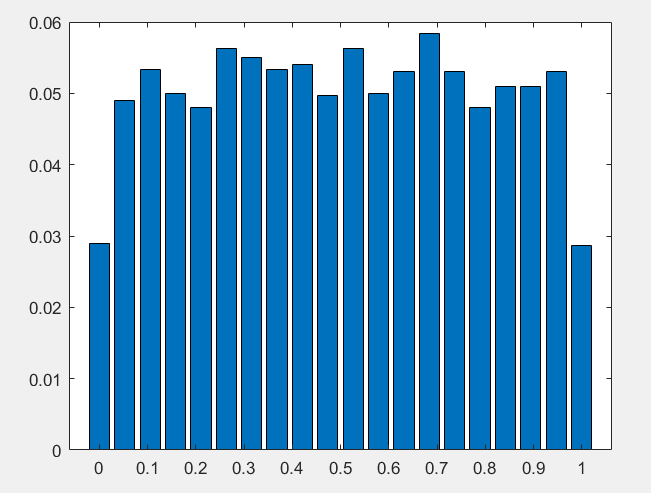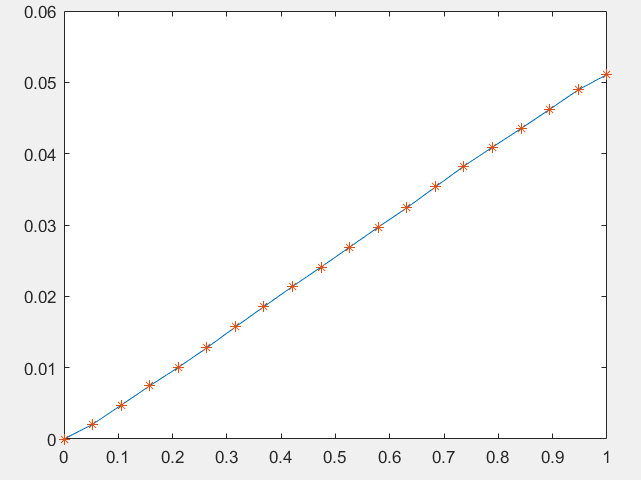clc;clear
x=randn(1,1000);
%Fr: 用matlab画出概率密度分布图
%//PDF的作用是用于计算概率，by 区间积分的方法
[mu,sigma] = normfit(x)
d=pdf('norm',x,mu,sigma);%概率密度函数，x对应的d
figure
plot(x,d,'.')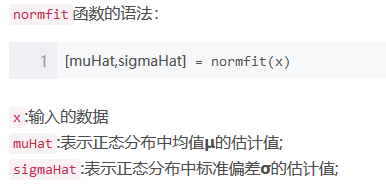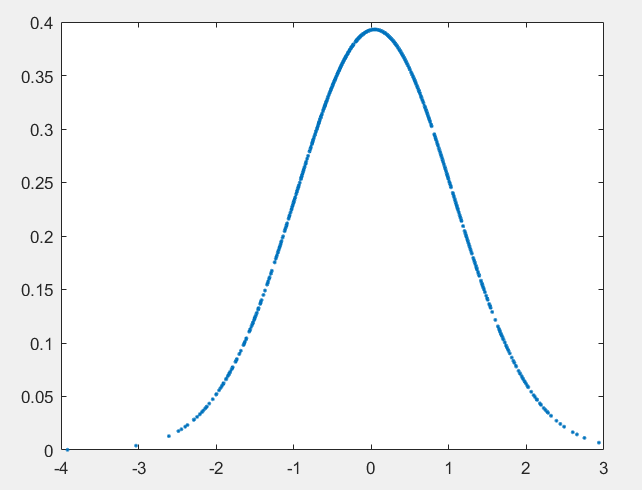展开全文matlab
• 本文你主要讲解了随机变量，分布函数的定义及基本性质；离散型随机变量的二项分布和泊松分布；连续型随机变量的定义，概率密度的性质，以及主要的均匀分布，正态分布，标准正态分布，Γ分布和指数分布
• 假设随机变量服从不同的分布，则可以求取该分布概率密度函数的参数。频率学派认为该参数是固定的，因此产生了最大似然估计。而贝叶斯学派认为该参数也是随机变量，产生了贝叶斯学习的方法。 1.1最大似然估计 *...
• bar(x,yy)%画出概率密度分布图 s=0 ; for i =2:length(x) s=[s,trapz(x([1:i]),yy([1:i]))] ; % please remove the " ; " end figure; plot(x,s,x,s,'*') Fr:  用matlab画出概率密度分布图   //...
• 在这一讲当中，我们再次看一种比较比较常见，适用情形非常固定的聚类算法，叫做混合模型，这种聚类算法是假设样本分布来自一个潜在的概率分布。或者若干个概率分布的混合。那么样本点的出现也就可以理解为从这个混合...算法
• 今天突然看到概率分布概率密度函数等概念，有点懵，赶紧复习以下。 理解相关概念首先要区分的是变量类型，离散变量与连续变量，不同的变量对应不同的概率描述方法，我们分开来看。 离散变量 概率分布概率密度是...
• 本篇文章是在《应该如何理解概率分布函数和概率密度函数？》的基础上整理来的。非常感谢原作者。 目录 1先从离散型随机变量和连续性随机变量说起 2离散型随机变量的概率函数，概率分布分布函数 2.1概率函数和...概率论与数理统计
• 概率密度和概率分布的区别 首先要理解概率密度函数，就要先分清离散型随机变量和连续型随机变量。 离散型随机变量，例如骰子的点数，取值只可能是1-6。连续型随机变量，例如温度，小数点后可能有很多位，是无法精确...机器学习 统计学
• 概率密度分布函数 联合概率密度 边际概率密度 条件概率密度 复合函数概率密度数据分析
• 概率密度函数是概率论中的核心概念之一，用于描述连续型随机变量所服从的概率分布。 从随机事件说起 回忆我们在学习概率论时的经历，随机事件是第一个核心的概念，它定义为可能发生也可能不发生的事件，因此是否发生...
• 概率密度估计方法用于估计一组数据集的概率密度分布，分为参数估计方法和非参数估计方法。 参数估计方法 假定样本集符合某一概率分布，然后根据样本集拟合该分布中的参数，例如：似然估计，混合高斯等，由于参数估计...
• matlab 二维 正态 概率密度 二维概率分布
• 　概率密度函数：用于直观地描述连续性随机变量（离散型的随机变量下该函数称为分布律）， 表示瞬时幅值落在某指定范围内的概率，因此是幅值的函数。连续样本空间情形下的概率称为 概率密度，当试验次数无限增加，...
• 概率分布函数（又称分布函数） 累积 F(x) = P{X<x}, x∈(﹣无穷，+无穷) 2.概率分布律(又成分布律，针对离散型变量) ...3.概率密度函数（又称密度函数，针对连续性变量） 累积 F(x)=∫f(t)dt ...机器学习
• PDF：概率密度函数（probability density function）, 在数学中，连续型随机变量的概率密度函数（在不至于混淆时可以简称为密度函数）是一个描述这个随机变量的输出值，在某个确定的取值点附近的可能性的函数。...概率统计
• 离散型随机变量的概率分布 对于离散型随机变量，设 为变量X的取值，而 为对应上述取值的概率，则离散型随机变量X的概率分布为 且概率 应满足条 。因此，离散型随机变量X的概率分布函数为 连续...
• 1、用到的时候总结一下，回过来...3、概率密度函数（probability density function）和概率分布函数（Cumulative Distribution Function，累积密度函数）。通过讲解正态分布去理解。 可以参考一下资料：https://zhuanl
• 1）概率密度函数是不是和分布律类似代表随机变量的概率值？ 2）如何通过样本数据估算总体的概率密度分布
• 数学基础复习之概率论（大部分来自百度百科和课本内容） ...在数学中，连续型随机变量的概率密度函数（在不至于混淆时可以简称为密度函数）是一个描述这个随机变量的输出值，在某个确定的取值点附近的可能性的
• （博客上编辑公式很麻烦，大多上自己文档的截图了） （随机试验，样本空间等可...2. 概率分布函数和概率密度函数（PDF） （概率分布函数针对离散和连续型随机变量，概率密度函数只针对连续型随机变量）随机变量
• pdf：连续随机分布概率密度函数 pmf：离散随机分布概率密度函数 cdf：累计分布函数 百分位函数（累计分布函数的逆函数） 生存函数的逆函数（1 - cdf 的逆函数） 函数里面不仅能跟一个数据，还能跟一个数组。...python 逆函数 scipy
• 概率函数：用函数形式给出每个取值发生的概率，P(x)(x=x1,x2,...)。只对离散型数据有意义。...概率密度函数：给出了xi落在某值x邻域内的概率变化快慢，概率密度函数的值不是概率，而是概率的变化率。概率密度
• 1.概率密度函数 　1.1. 定义 　如果对于随机变量X的分布函数F(x),存在非负函数f(x),使得对于任意实数有 　则称X为连续型随机变量，其中F(x)称为X的概率密度函数，简称概率密度。（f(x)>=0,若f(x)在点...
• 随机变量的分布函数： 1. 定义 设X是一个随机变量，x是任意实数，函数F(x)=P{X 2.1 性质 对于任意x1、x2...随机变量的密度函数： 1. 定义 如果对于随机变量X的分布函数F(x),存在非负函数f(x),使得对于任意实数...